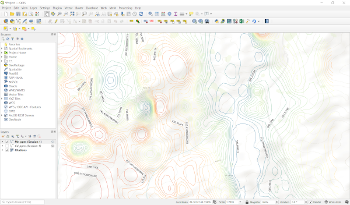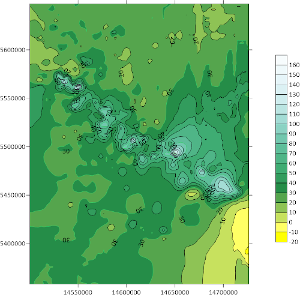1. A mathematical model of the geochemical problem has been developed in the form of an inverse problem of the continuation of potential fields towards disturbing masses using the mathematical apparatus of integral equations. The problem is to solve the Fredholm integral equation of the first kind for a large number of different right-hand sides, while the core of the integral equation remains the same. Mathematical models, methods and algorithms for solving the inverse problem based on geophysical data (vector fields) have been developed. A mathematical model of the coefficient inverse problem of inhomogeneous geochemical fields based on the Gelfand-Levitan equation has been developed.

2. A mathematical model of the tomography problem based on geochemical data based on the representations of statistical physics has been developed. The developed methods of numerical realization of inverse problems of mathematical geophysics and geochemistry have shown satisfactory accuracy on test examples.

3. Algorithms for numerical solution of Fredholm integral equations of the first kind have been developed. A new method for the numerical solution of these equations based on the application of the theory of conjugate equations is proposed. A method of numerical solution of Fredholm integral equations of the first kind by projection methods with bases in the form of Legendre wavelets has been developed. The questions of mathematical substantiation of the proposed methods are investigated. Numerical calculations of test problems were carried out.4. A software module has been developed for the QGIS system version 3.18.3-Zurich, the functioning of which is based on the application of the theory of inverse problems of mathematical geophysics using geological data on the earth's surface, geophysical measurements and geochemical analyses as input data. The software module is based on a complex predictive-mineragenic model consisting of geochemical and geophysical parts and digital modeling by methods of inverse problems of geochemistry and geophysics, which is aimed at the regional geological study of the subsurface, namely the deep parts of the Earth's crust.

The software module provides users of the QGIS system with a set of methods for reconstructing the distribution fields of chemical elements at a given depth based on mathematical modeling, and has the following functionality:

a) Import of measurement input data from CSV format files into the database;

b) Carrying out predictive calculations based on the input measurement data and the algorithm for solving the inverse problem;

c) Visualization of the initial data and data obtained as a result of solving the inverse problem.5. The numerical data were processed and analyzed using the above mathematical methods and a set of application programs. The data were obtained in the engineering laboratory during analytical studies of ICP-MS spectroscopy, 777 samples taken on 70 chemical elements in the surveyed area of over 40 thousand square kilometers, which were obtained in expeditionary studies on geochemistry and mineralogy of loose deposits of mineral deposits of the Rudny Altai and Kalba.